# Java code examples to learn how to program easily and quickly

Are you just starting to learn programming and are looking for Java code examples? You can stop your search now, today we are going to tell you some examples that will be very useful for your development projects.

But first, do you know what Java is? it is a programming language that allows you to write commands, computer instructions and lines of code in a way that can be easily understood and read by humans. And, according to the Java site, this language “is fast, secure and reliable”.

Java is intended for the development of an infinite number of applications: from dynamic sites to applications for mobile devices. The reality is that there is an extensive list of options and tools that we can develop in Java. that is why learning Java coding is important in the market for new software development, as it is one of the most widely used programming languages today.

The best way to learn Java coding is through examples. By internalizing the codes and understanding the operation and usefulness of this programming language, we will be able to explore the full potential of our Java codes. Remember that, as the saying goes, “practice makes perfect”.

that is why we prepared this post in which we will review simple examples of Java code. Computer coming soon? Join us!

## Hello World

Among all the simple Java programs, this is the first one you should learn. it is the first Java code that almost all programmers compile. Here is your code: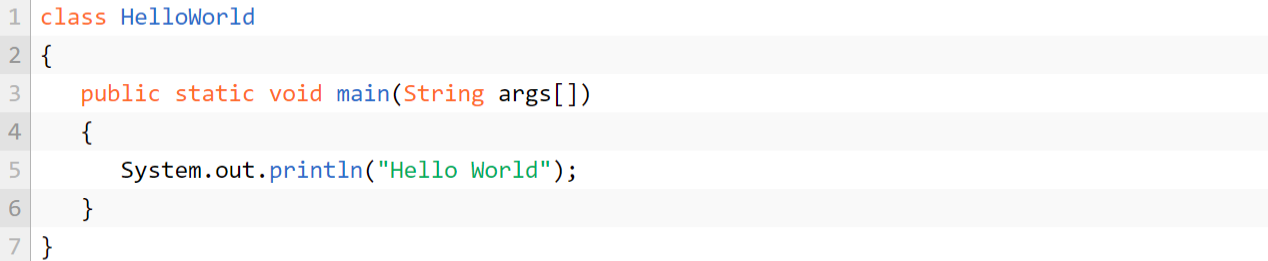Source: Javatutoring

### Points to be considered

The class definition (class): it is used to define a new class through the keyword “class” in Java codes. It must be signed with keys: opening “{” and closing “}” in Java codes.
Main method (main): every Java application must contain the method, in this case, the public static void main (String [ ] args)
Public: it is placed so that it can be executed anywhere.
Static: with this the main method can be called without creating the object.
Void: the main method returns nothing.
String: the main method accepts a single argument.

## area of a circle

The following Java code will be very useful for you, so we recommend that you pay close attention to it.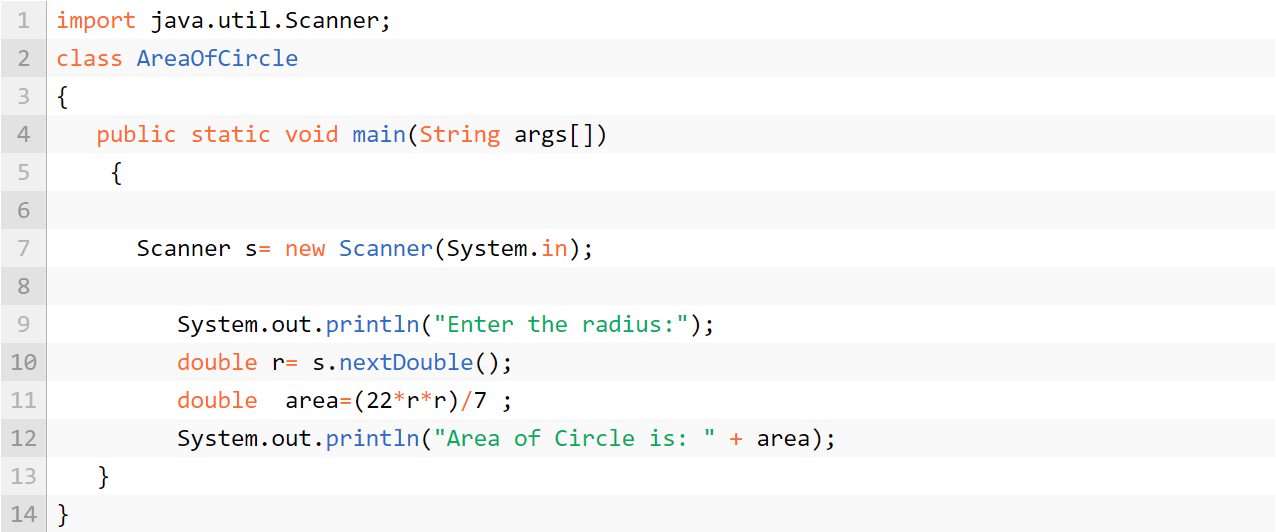Source: Javatutoring

### Points to be considered

To obtain the area of a circle, we must define the formula that, as we know, consists of A = r2 π.
In addition, we attribute the value of 22/7 to the Greek symbol for “pi”.
The “Import” is a keyword with which we can obtain certain characteristics of the integrated packages. Here we use the “util” package which includes many classes. One of these classes is “Scanner”, which we use to get commands through the console. Let’s remember that the console is the interface between the user and the program.
Next, we can see the main function that we already explained in details in “public static void main”.
The scanner class is responsible for digitizing the input data provided by the user through the console. We must create an object that is the reference to store the variable “s”. (To access the console, we must create an object “new scanner “).
Now with System.out.println (“Enter the radius:”); we ask for the value of the radius that the user enters by keyboard.
We use the “nextdouble”, because this is the format we need for the console to read the number we are inserting. Besides, as the number of pi is a number with decimals, it is necessary to use “nextdouble” instead of “nextint” (a format that does not accept decimals, but only integers).

## triangle area

If you want to solve the area of the triangle with Java, we must create a class, and inside this class place a main method. Let’s see how these lines of code would look like in Java: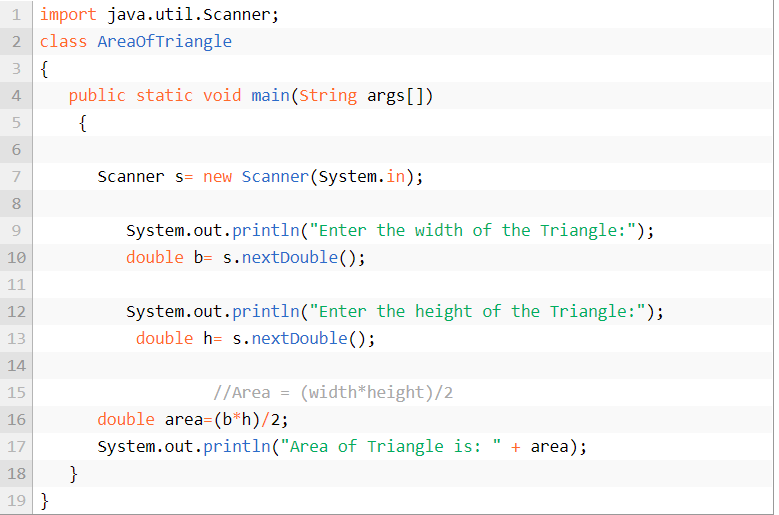Source: Javatutoring

### Points to be considered

For the case of the area of a triangle, we could say that its Java code is very similar to that of the area of a circle. It has a scanner to read the entries by console and we use “nextdouble”, once the inserted values can be decimal, as in the previous case.
It will be enough to ask for values such as height and base of the triangle by console to obtain its area, using the formula Atri = (base * height)/2.

## area of an isosceles triangle

How can we obtain the area of isosceles triangles? Let’s see this next Java code!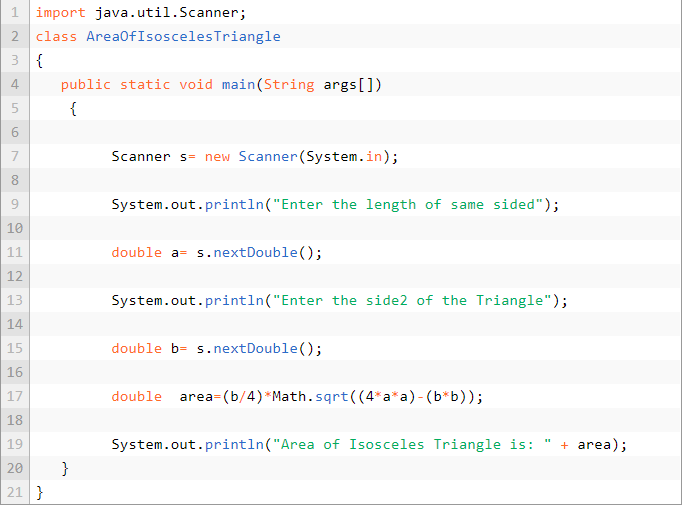Source: Javatutoring

## area of an equilateral triangle

What if we wanted an equilateral triangle?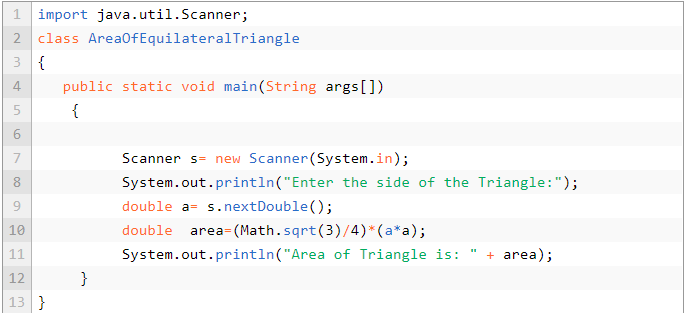Source: Javatutoring

## angle area

Now, let’s see what the area of the angle would be like in Java: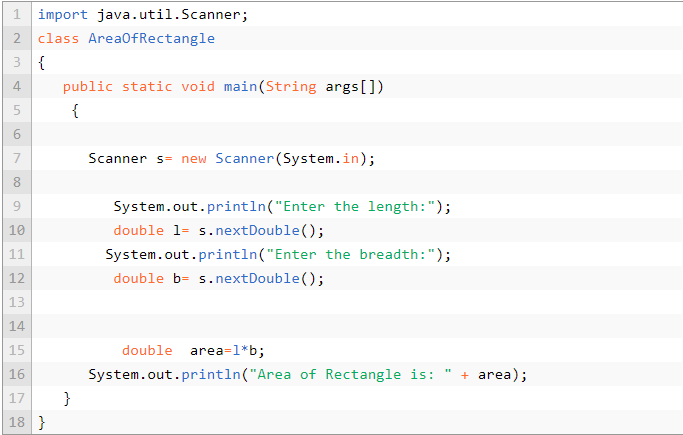Source: Javatutoring

### Points to be considered

Finally, and within the flat geometric figures, for the area of the angle we will follow the same parameters: a class, the main block composed by “public static void main”, a scanner and the next nextdouble format.
This way, the Java code will print the area of the angle on the canvas following the mathematical formula of A = base * height.

## Perimeter of the rectangle

What if, instead of the area, we try to calculate the perimeter of the rectangle with the Java code?

Basically, the variation of the Java code is in the formula. The formula is defined by P = 2(base * height), as seen below: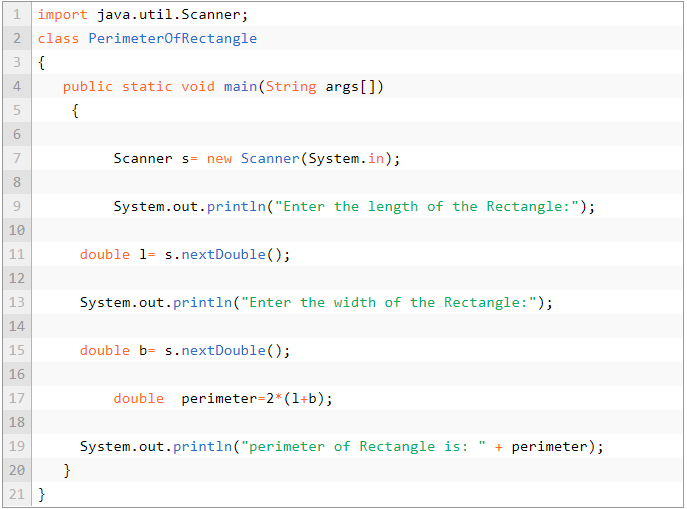Source: Javatutoring

## Perimeter of a square

In the case of a rectangle, we need to know both the base and the height. In this case, the sides of a square have all the same size, therefore, knowing the size of the side will give us the perimeter. See the Java code: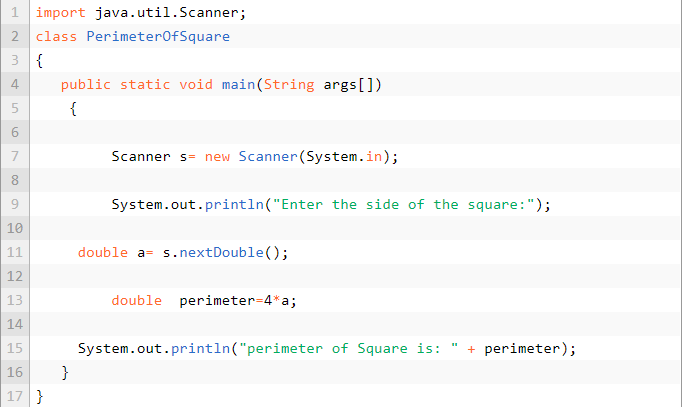Source: Javatutoring

## Volume of a sphere

Up to now, in Java codes, we have worked with areas of flat surfaces, but we can also find volumes in solid surfaces.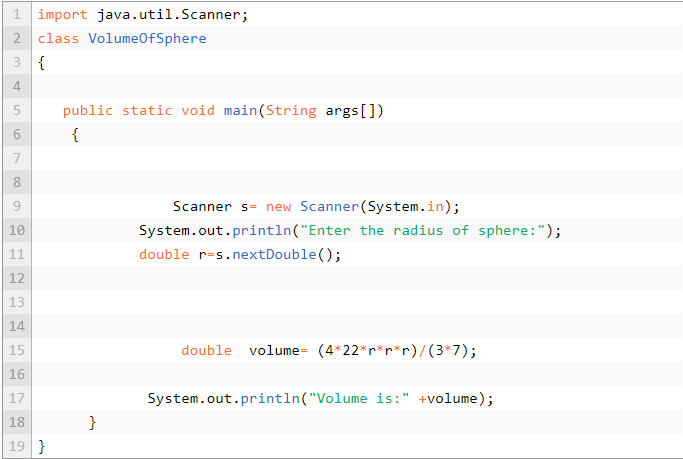Source: Javatutoring

### Points to be considered:

Embora estejamos falando de uma superfície sólida, o procedimento ainda é bastante semelhante. The first line of code still contains the “import”, to obtain certain characteristics and embedded packages. In the same way, in this case, we still use the “util” package and the “nextdouble” format.
In conclusion, the only thing that changes is the formula used and the data needed to obtain the result you see on the screen.

And just as we obtain complex areas like a sphere, we can find values for many solid or flat surfaces: hexagons, pentagons, cylinders, etc.

## Calculate the average of “n” numbers

Whenever we have a numerical value, we must enter a formula to the code. In this case, we say that the average of the “n” numbers is: the sum of all values / the number of values.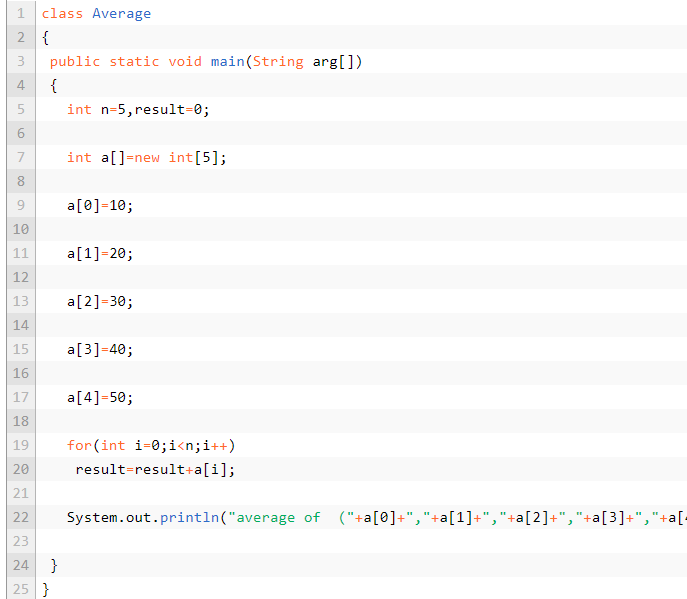Source: Javatutoring

### Steps to be considered

We start by assigning a name and a class and start the algorithm with “public static void main”.
In this case, we define the variable “n” under the format “int”, since the numbers to work with are integers.
Subsequently, in line 12 we can see how we attribute, we ask the user to enter the amount of numbers for which we will calculate the average.
Our next step will be based on the cycle “for”, to add the numbers.
Finally, once we have inserted all the numbers, our program will be executed and will display the average of the inserted numbers.

## Calculate the average of beats

Modifying a little bit the case of the average, we can see how to calculate the average of a batcher through the Java code: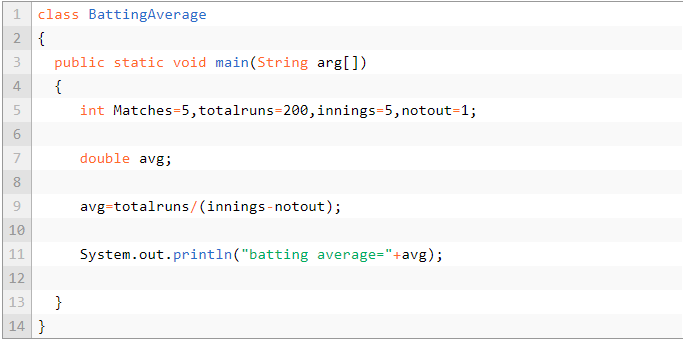Source: Javatutoring

Continuing the trend of simpler Java codes, we can continue with the addition of only two numbers. For this, we use the following code: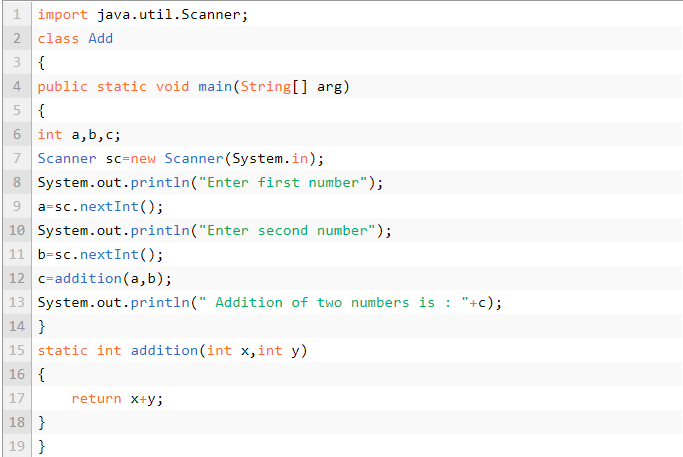Source: Javatutoring

### Points to be considered

As in previous cases, we define name and class. We start the code and define 3 variables (two that we will request to the user and one will be the result of the previous ones).
The user registers the numbers and the program executes the command.

Now, what happens if instead of 2 numbers, I want to make the soma of a quantity “n” of symbols”?

Have you already had an idea of how to generate the same algorithm but, instead of 2 numbers, add the desired amount? Maybe you already know the answer (because we did a similar algorithm before). If your answer is that it is a cycle, you are right. that’s how it’s done: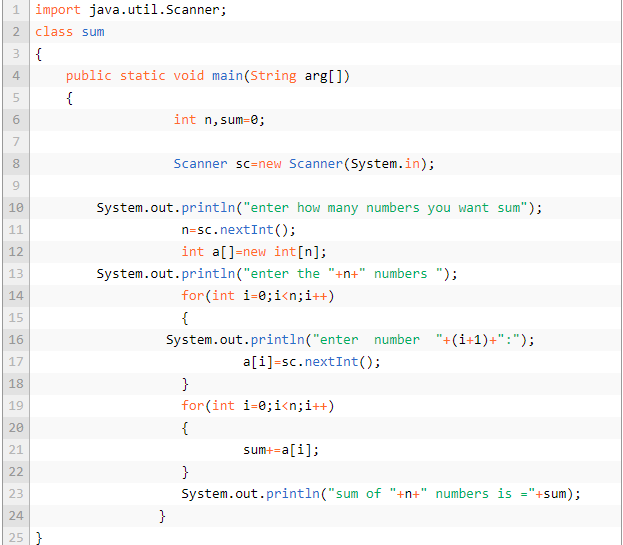Source: Javatutoring

## Calculate the electricity bill

Another tool that we can develop through the Java code is the electricity bill. We show the code procedure in the image below: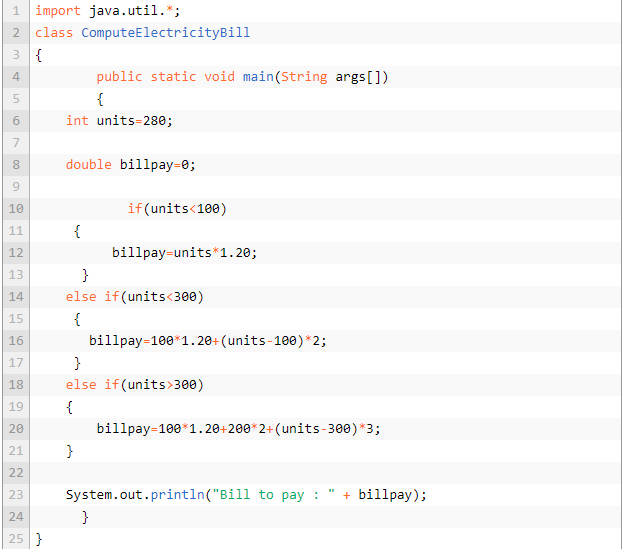Source: Javatutoring

Points to be considered:

The only difference between this and the other Java codes we have already studied, is that we add the “if” and ” if else” conditions.
These lines will be executed, whenever a condition executed by the user is fulfilled.

## Calculate the discount of a product

sometimes, we want to know the discount of a product to obtain its final product. For this, we can use a simple Java code that facilitates this calculation in a matter of seconds. Below, we show how this is done:Source: Javatutoring

Points to be considered

The only values that the code requires are the price of the item and its discount.

## Sixth year

Through the use of the conditions offered by “if”, it is shown the procedure to know if a year is a leap year or not. Remember that the objective is to obtain mental agility to create any algorithm that you want or need to do.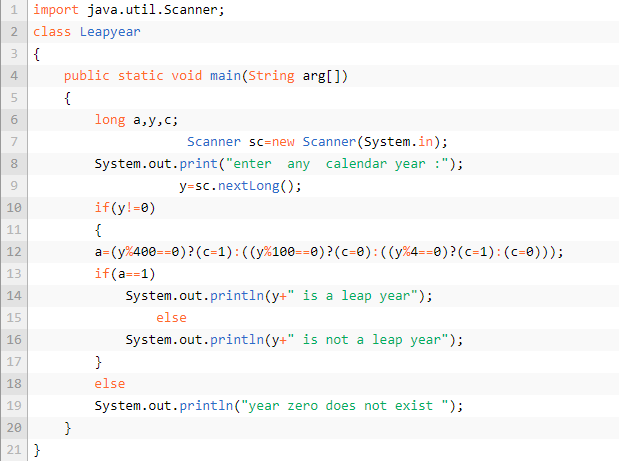Source: Javatutoring

## Obtain the distance between two points

The most “complex” (in fact it is not) in this particular case is to know the formula needed to perform this logarithm. Therefore, pay special attention to the formula used.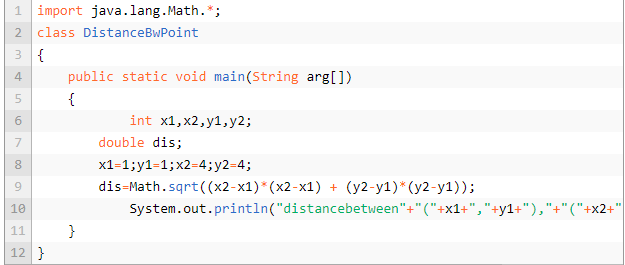Source: Javatutoring

Another example in this top of Java codes has to do with the world of matrices. These will meet certain requirements to be executed correctly:

They must have the same size and be inserted in the same order.
Besides the matrix, each term of one of them is added to the term of the other, in the same place. A bit awkward, isn’t it? Don’t worry, we will show you a graphic example of how it works to add with the Java code: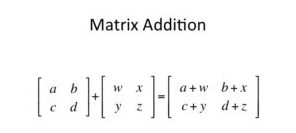Source: Javatutoring

Below is the overview of the matrices in Java code: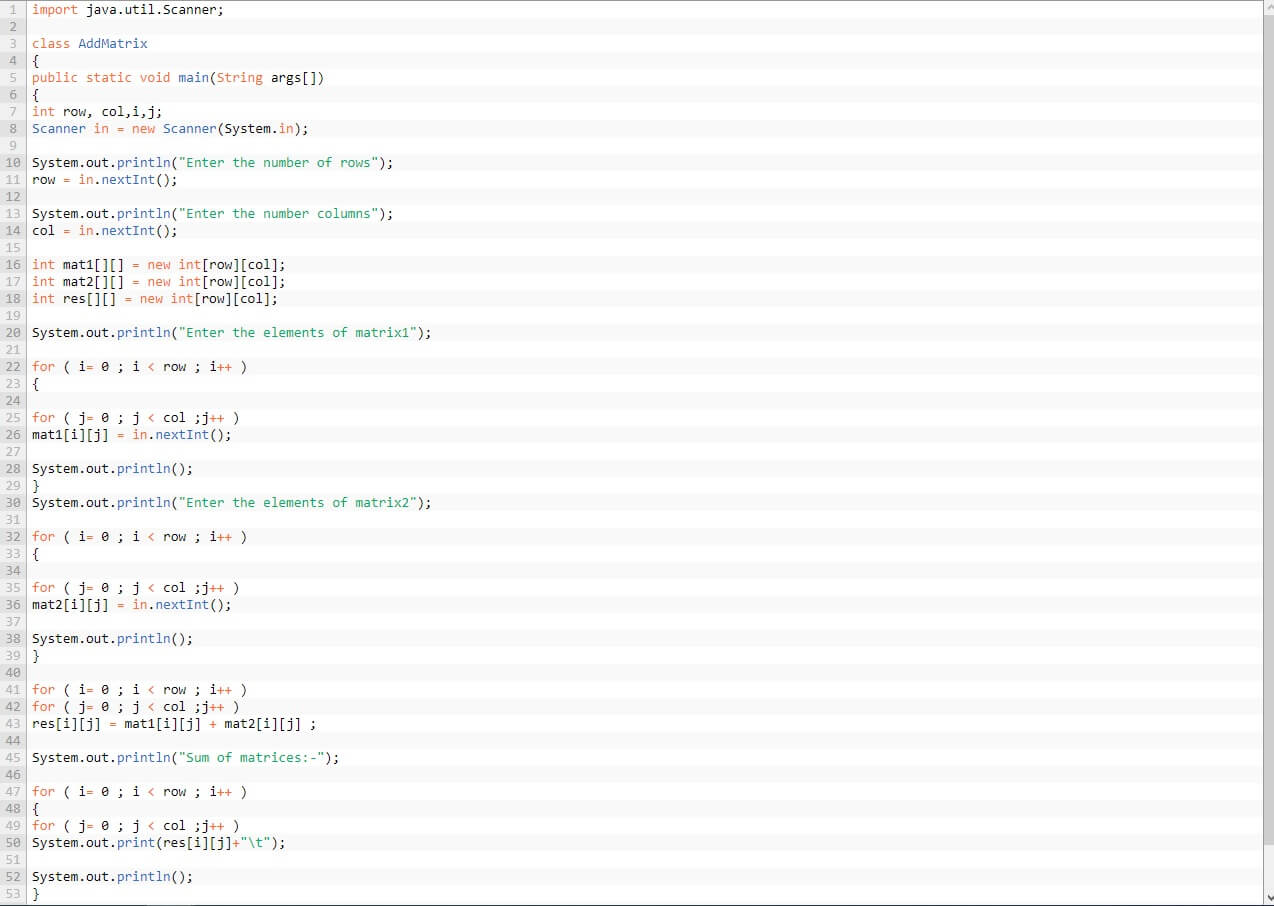Source: Javatutoring

## Subtraction of two matrices

In this case, matrix subtraction in Java must be done between two matrices of the same order. We demonstrate this graphically to facilitate its understanding: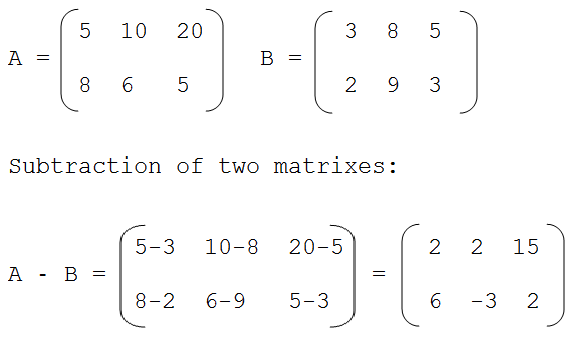Source: Javatutoring

On the other hand, the subtraction of the matrices is done as follows: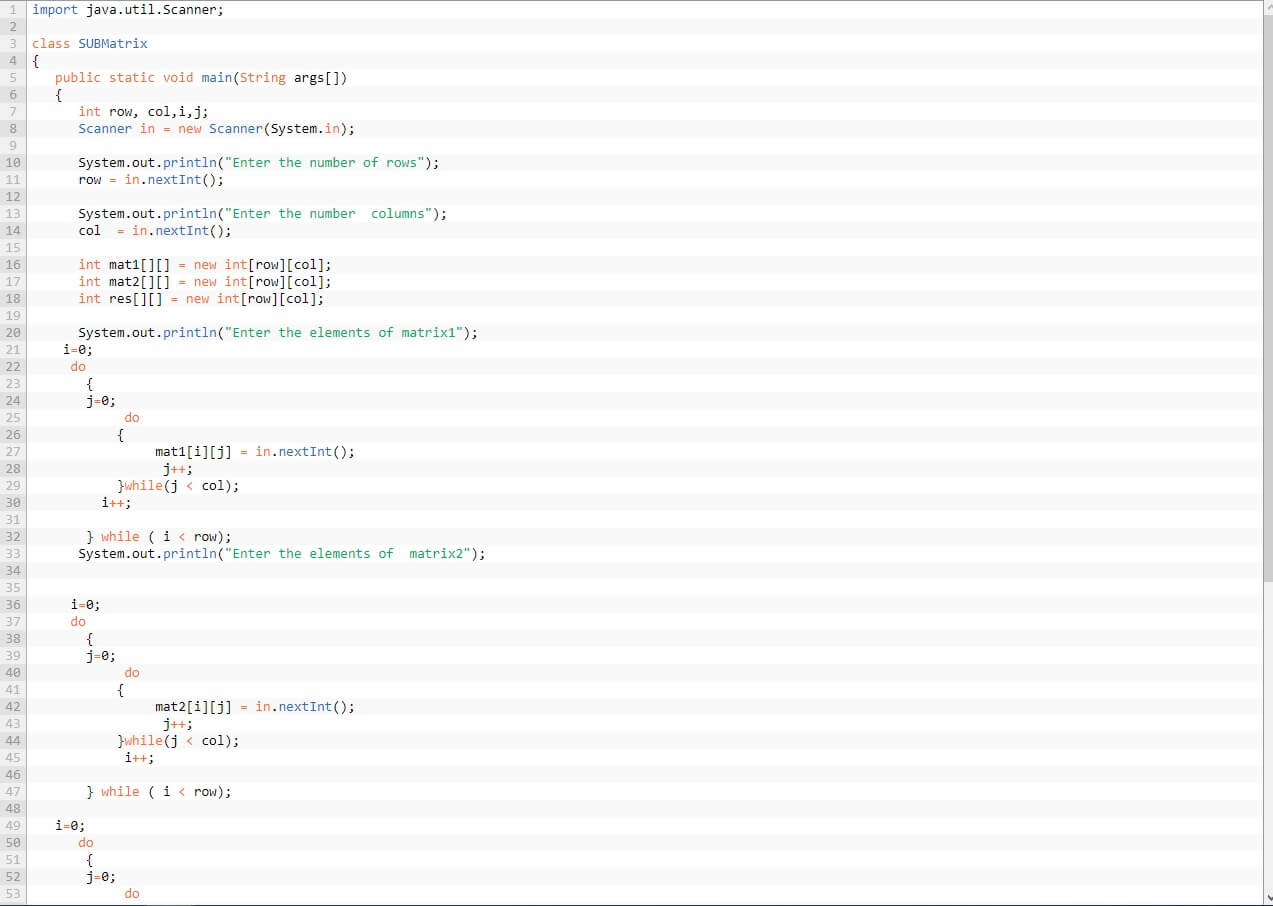Source: Javatutoring

## Matrix multiplication

In this other java code example, we can highlight the multiplication of matrices under the following code: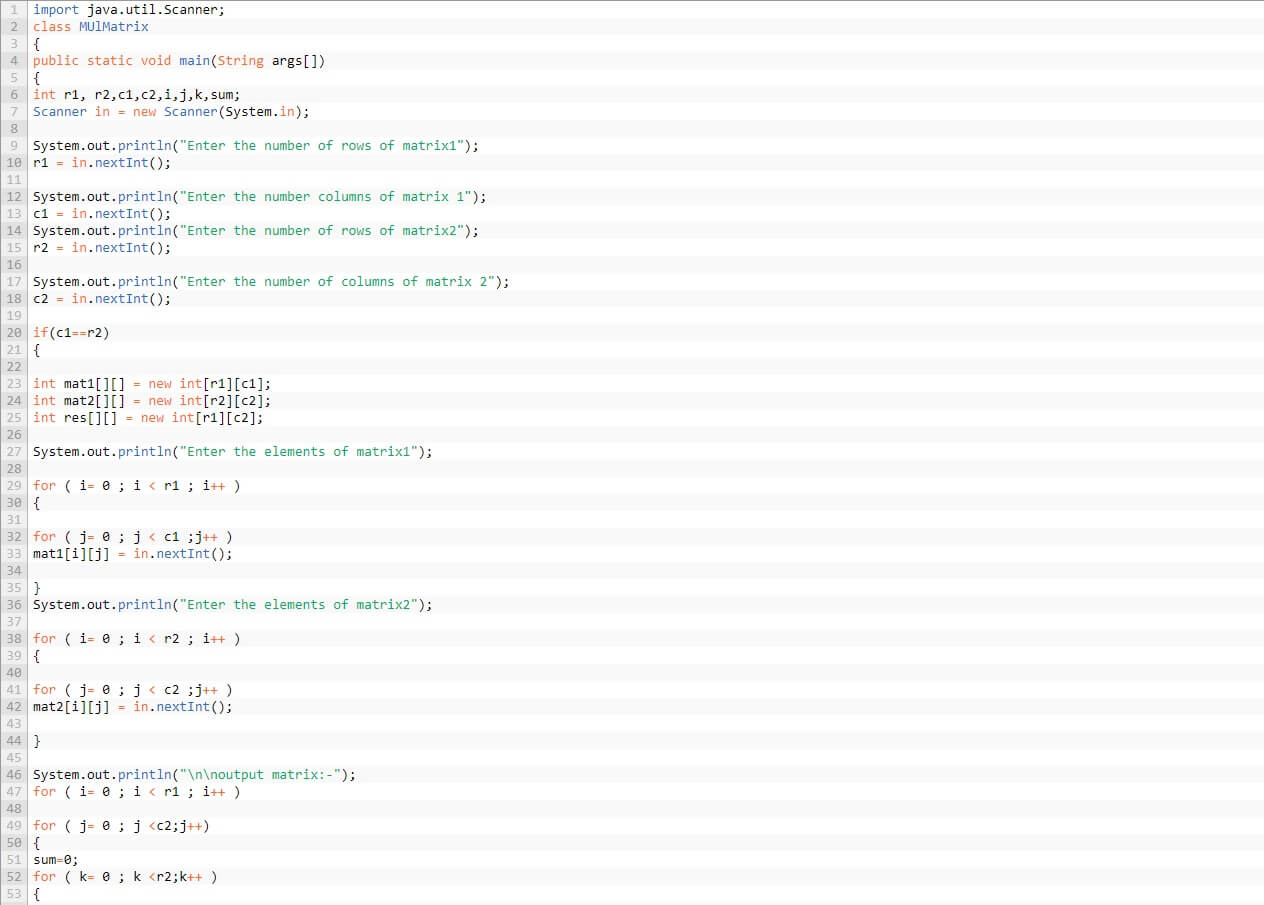Source: Javatutoring

## TableModel in JTable

Let’s see how to use a Java JTable. it is a visual component of Java that allows you to design a table and add data in each row and column.

Besides using other JTable builders, one of the easiest ways to use a JTable is to instantiate as a data model a DefaultTable and then a JTable, passing the model to the builder. The code would look like this:Image: chuidiang

Now you can add columns to our data with the Java code: datos.addColumn (“Nome coluna”);

And then you can add, turn off and modify data like this:

data.removeRow ( row );
data.setValueAt (data, line, column);

When you make modifications, the JTable will update the result automatically.

## Start an external program from Java

It may happen that some times you need to call external executables, for example: launch Acrobat Reader to view a pdf file from the browser. For these situations, this Java code example will help you a lot.

it is important to consider that this practice is not highly recommended, because it loses the whole objective of Java, which is to be able to run the program on any platform. However, it is sometimes necessary.

The code would look like this: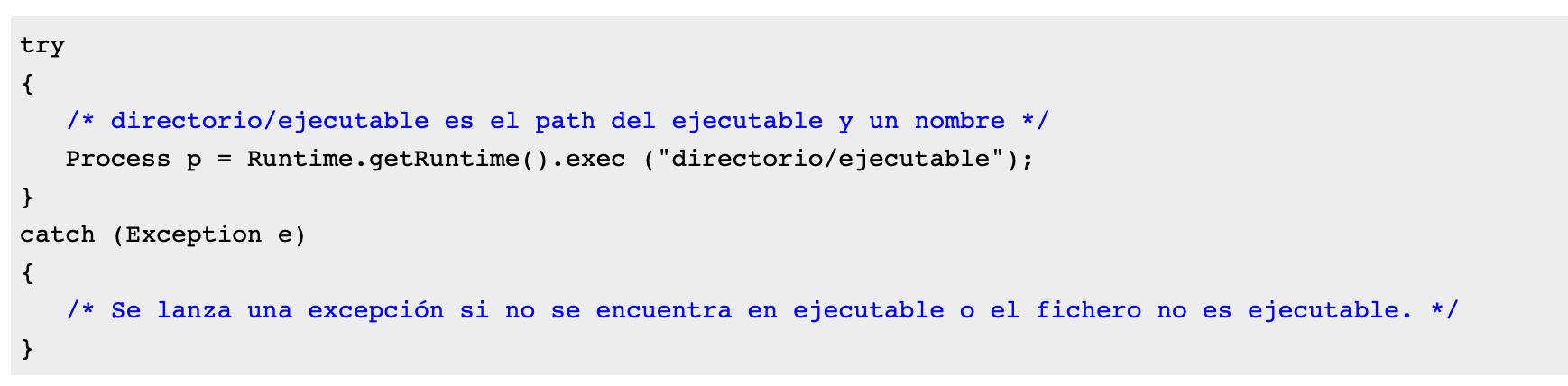Image: chuidiang

## Verify composite number

This Java code, proposed in the blog Java do Zero, allows you to verify if a number is composed. All you need to do is alter the return statements. The return true is changed to return false and vice versa. it is very simple!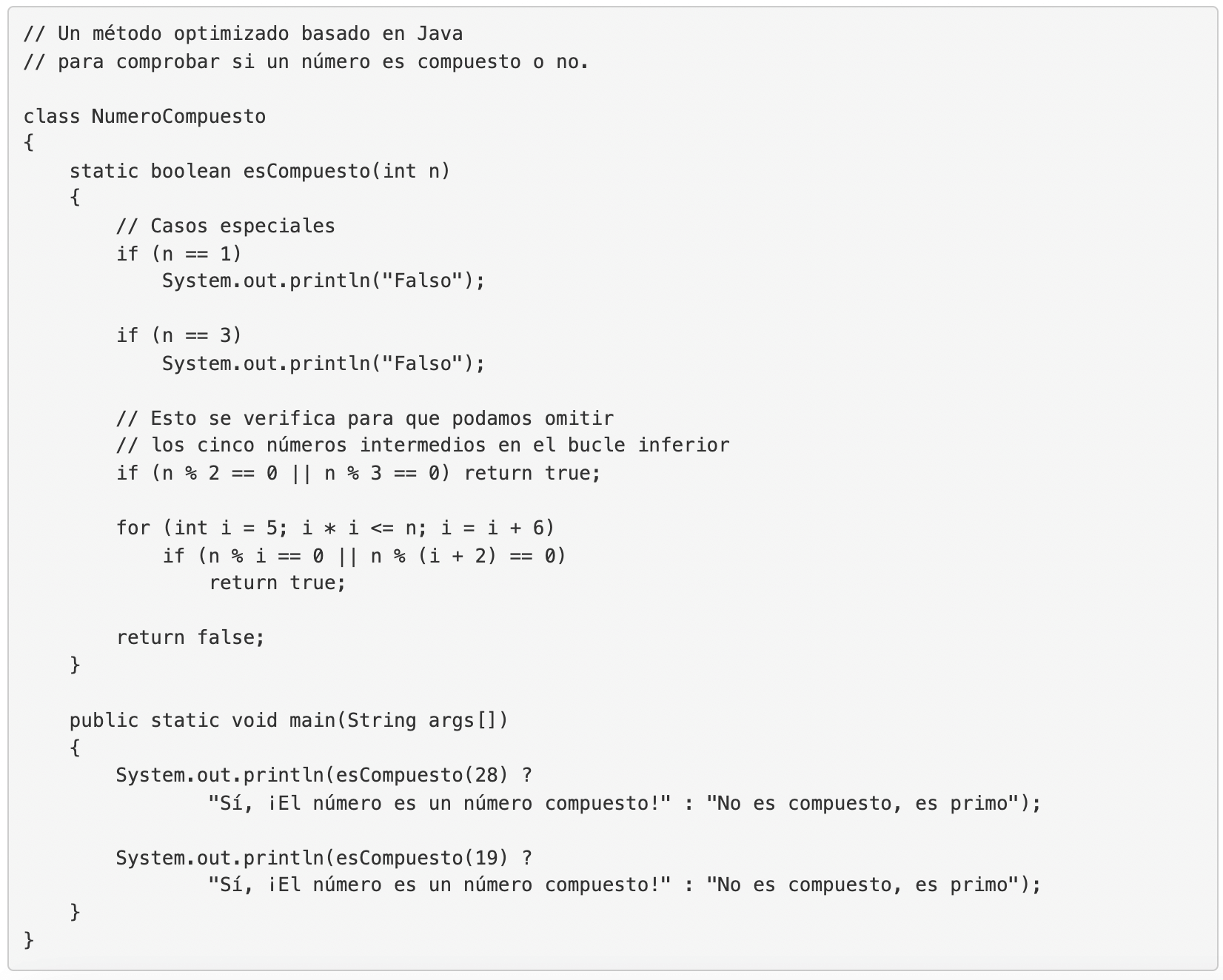Image: Java from scratch

The result of this Java code produces two results:

Yes, the number is a composite number!
It is not composite, it is prime

## Verify if a URL is valid

These Java codes were also published in the blog Java do Zero, and they are three methods to verify if a web address is valid or not.

The expected result of the three methods is: “The given url (url) is valid” or “The given url (url) is invalid”.

Next, we will tell you what these codes are for Java.

### Validating URLs with the Apache Commons validator

With the Apache Commons Validator package, you can use the URLValidator class to validate the URL, verifying the schema, authority, path, query and snippet. This is what the code would look like: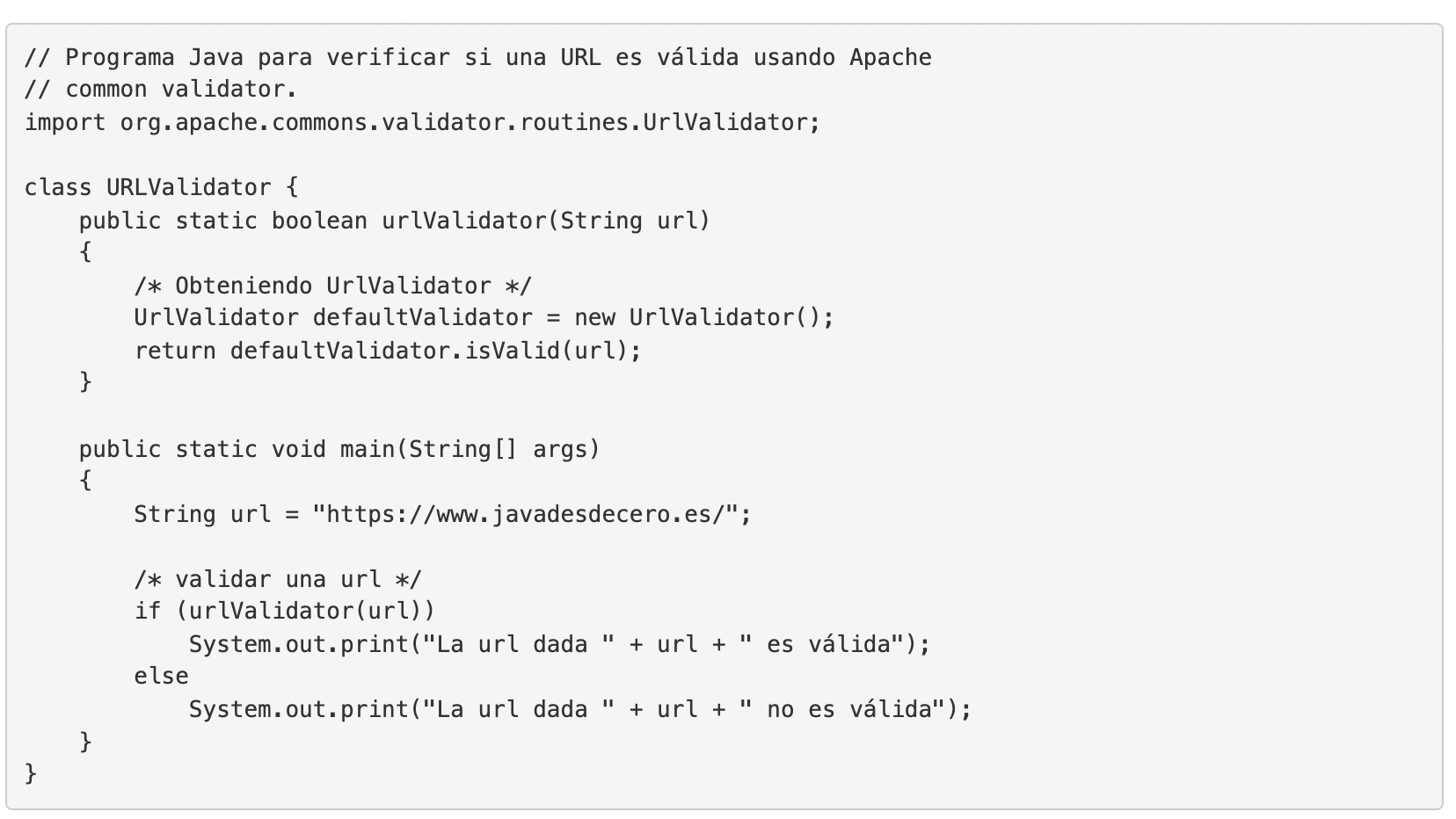Image: Java from scratch